# Venn diagrams with eulerr

#### 2020-03-09

Venn diagrams are specialized Euler diagrams. Unlike Euler diagrams, they require that all intersections are represented. In most implementations—including eulerr’s—they are also not area-proportional.

The first requirement is often prohibitive in terms of interpretability since we often waste a considerable amount of space on the diagram’s canvas with intersections that just as well might be represented by their absence. An area-proportional Euler diagram is often much more intuitive and, for relatively sparse inputs, much easier to interpret. The property of being area-proportional may sometimes, however, be treacherous, at least if the viewer isn’t advised of the diagram’s faults.

In such instances, it is often better to give up on area-proportionality and use a Venn diagram. It might not be as easy on the eye, but at least will be interpreted correctly.

eulerr only supports diagrams for up to 5 sets. Part of the reason is practical. eulerr is built around ellipses and ellipses are only good for Venn diagrams with at most 5 sets. The other part of the reason has to do with usability. A five-set diagram is already stretching it in terms of what we can reasonably expect the viewer to be able to decipher. And despite the hilarity of banana-shaped six-set Venn diagrams, monstrosities like that are better left in the dark.

## Examples of Venn and Euler diagrams

We will now look at some Venn diagrams and their respective Euler diagrams.

library(eulerr)
set.seed(1)

s2 <- c(A = 1, B = 2)

plot(venn(s2))
plot(euler(s2), quantities = TRUE)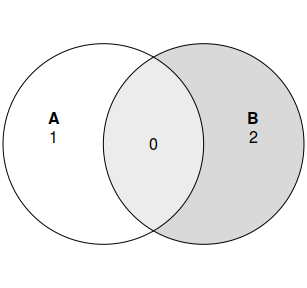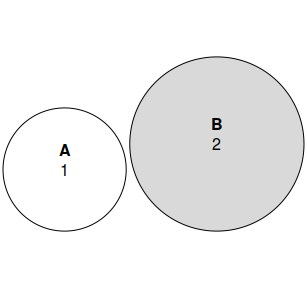plot(venn(fruits[, 1:3]))
plot(euler(fruits[, 1:3], shape = "ellipse"), quantities = TRUE)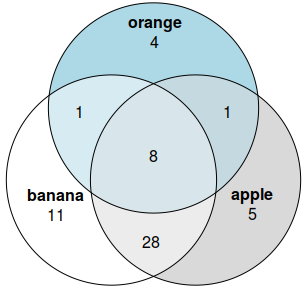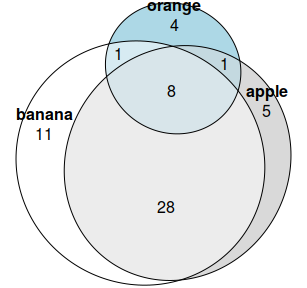s4 <- list(a = c(1, 2, 3),
b = c(1, 2),
c = c(1, 4),
e = c(5))
plot(venn(s4))
plot(euler(s4, shape = "ellipse"), quantities = TRUE)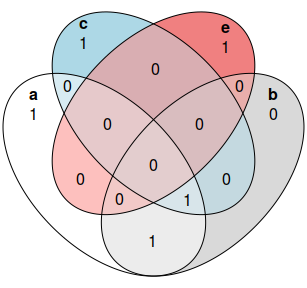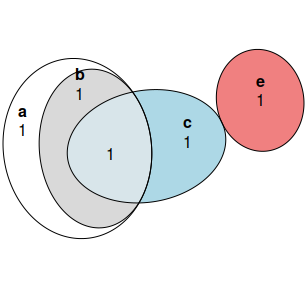plot(venn(organisms))
plot(euler(organisms, shape = "ellipse"), quantities = TRUE)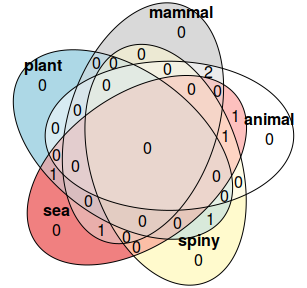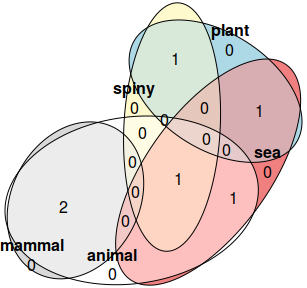As you can see in the last plot, there are cases where Euler diagrams can be misleading. Despite the algorithm attempting its best to make the diagram area-proportional, the constraints imposed by the geometry of the ellipses prevent a perfect fit. This is probably a case where a Venn diagram makes for a good alternative. In the author’s opinion, the opposite is true for the rest.